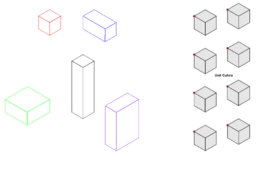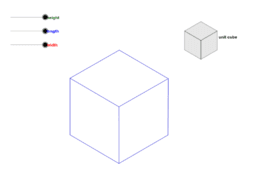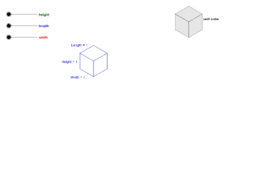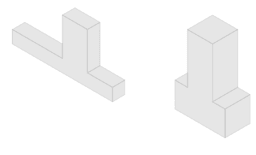# CCSS Gr. 5 Volume

Topic:
Geometry
Common Core Standards for Grade 5-Geometry Graph points on the coordinate plane to solve real-world and mathematical problems. 1. Use a pair of perpendicular number lines, called axes, to define a coordinate system, with the intersection of the lines (the origin) arranged to coincide with the 0 on each line and a given point in the plane located by using an ordered pair of numbers, called its coordinates. Understand that the first number indicates how far to travel from the origin in the direction of one axis, and the second number indicates how far to travel in the direction of the second axis, with the convention that the names of the two axes and the coordinates correspond (e.g., x-axis and x-coordinate, y-axis and y-coordinate). 2. Represent real world and mathematical problems by graphing points in the first quadrant of the coordinate plane, and interpret coordinate values of points in the context of the situation. Classify two-dimensional figures into categories based on their properties. 3. Understand that attributes belonging to a category of two-dimensional figures also belong to all subcategories of that category. For example, all rectangles have four right angles and squares are rectangles, so all squares have four right angles. 4. Classify two-dimensional figures in a hierarchy based on properties. Common Core Standards for Grade 5-Geometric Measurement Geometric measurement: understand concepts of volume and relate volume to multiplication and to addition. 3. Recognize volume as an attribute of solid figures and understand concepts of volume measurement.
• A cube with side length 1 unit, called a “unit cube,” is said to have “one cubic unit” of volume, and can be used to measure volume.
• A solid figure which can be packed without gaps or overlaps using n unit cubes is said to have a volume of n cubic units.
4. Measure volumes by counting unit cubes, using cubic cm, cubic in, cubic ft, and improvised units. 5. Relate volume to the operations of multiplication and addition and solve real world and mathematical problems involving volume.
• Find the volume of a right rectangular prism with whole-number side lengths by packing it with unit cubes, and show that the volume is the same as would be found by multiplying the edge lengths, equivalently by multiplying the height by the area of the base. Represent threefold whole-number products as volumes, e.g., to represent the associative property of multiplication.
• Apply the formulas V = l × w × h and V = b × h for rectangular prisms to find volumes of right rectangular prisms with whole-number edge lengths in the context of solving real world and mathematical problems.
• Recognize volume as additive. Find volumes of solid figures composed of two non-overlapping right rectangular prisms by adding the volumes of the non-overlapping parts, applying this technique to solve real world problems.
•### VOLUME AND THE UNIT CUBE

•### ESTIMATE VOLUME

•### DISCOVERING A WAY TO COMPUTE VOLUME

•### COMBINED VOLUMES

•### More Combined Volumes

•